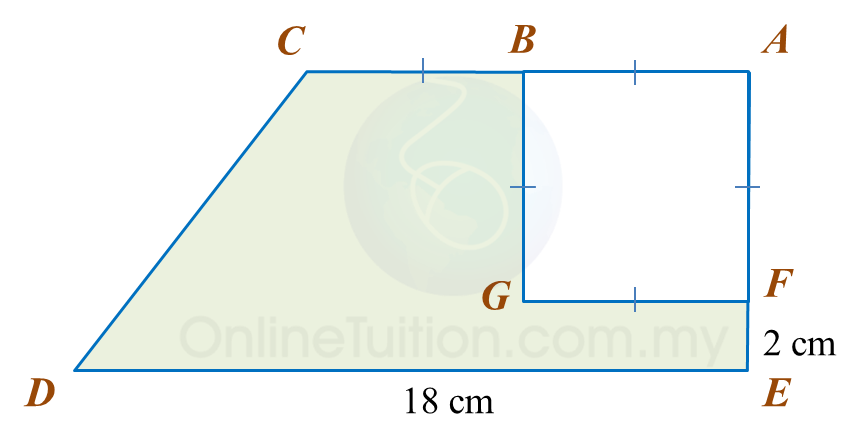# 10.2.3 Perimeter and Area, PT3 Practice

Question 7:
In diagram below, AEC is a right-angled triangle with an area of 54 cm2 and BCDF is a rectangle.Calculate
(a) the perimeter, in cm, of the coloured region.
(b) the area, in cm2, of the coloured region.

Solution:

Question 8:
Diagram below shows a trapezium ABCDE. ABGF is a square with an area of 36 cm2.Calculate
(a) the perimeter, in cm, of the coloured region.
(b) the area, in cm2, of the coloured region.

Solution:### 1 thought on “10.2.3 Perimeter and Area, PT3 Practice”

1.Terima kasih banyak banyak